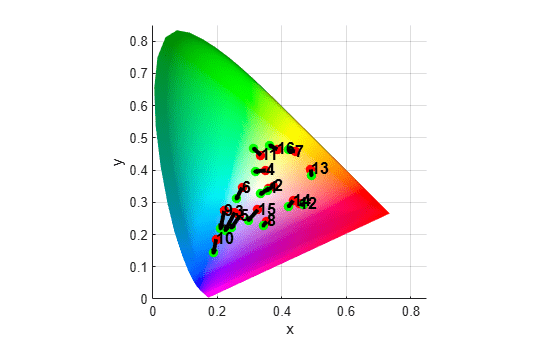# plotChromaticity

Plot color reproduction on chromaticity diagram

## Syntax

``plotChromaticity(colorTable)``
``plotChromaticity``
``plotChromaticity(___,Name,Value)``

## Description

example

````plotChromaticity(colorTable)` plots on a chromaticity diagram the measured and reference colors, `colorTable`, for color patch regions of interest (ROIs) in a test chart.```
``plotChromaticity` plots an empty chromaticity diagram.`
````plotChromaticity(___,Name,Value)` adjusts aspects of the display using name-value arguments.```

## Examples

collapse all

This example shows how to display the chromaticity diagram from measurements of color accuracy on an Imatest® eSFR chart.

Read an image of an eSFR chart into the workspace.

`I = imread('eSFRTestImage.jpg');`

Create an `esfrChart` object. Display the chart, highlighting the 16 color patches.

```chart = esfrChart(I); displayChart(chart,'displayEdgeROIs',false, ... 'displayGrayROIs',false,'displayRegistrationPoints',false)```Measure the color in all color patch ROIs.

`colorTable = measureColor(chart);`

Plot the measured and reference colors in the CIE 1976 L*a*b* color space on a chromaticity diagram. Red circles indicate the reference color and green circles indicate the measured color of each color patch. The chromaticity diagram does not portray the brightness of color.

```figure plotChromaticity(colorTable)```Read an image of a ColorChecker® chart into the workspace.

`I = imread("colorCheckerTestImage.jpg");`

Create a `colorChecker` object, then display the chart with ROI annotations.

```chart = colorChecker(I); displayChart(chart)```Measure the color in each color patch ROI.

`colorTable = measureColor(chart);`

Plot the measured and reference colors on a chromaticity diagram.

```figure plotChromaticity(colorTable)```Convert sRGB primary colors to the XYZ color space.

`xyz_primaries = rgb2xyz([1 0 0; 0 1 0; 0 0 1]);`

Normalize the x and y values of the primary colors.

```xyzMag = sum(xyz_primaries,2); x_primary = xyz_primaries(:,1)./xyzMag; y_primary = xyz_primaries(:,2)./xyzMag;```

Calculate and normalize the D65 white point.

`wp = whitepoint('D65');`

Normalize the x and y values of the white point.

```wpMag = sum(wp,2); x_whitepoint = wp(:,1)./wpMag; y_whitepoint = wp(:,2)./wpMag;```

Create an empty 2-D chromaticity diagram.

`plotChromaticity`

Add the (x,y) coordinates of the primaries and white point to the chromaticity diagram.

```hold on scatter(x_whitepoint,y_whitepoint,36,'black') scatter(x_primary,y_primary,36,'black') plot([x_primary; x_primary],[y_primary; y_primary],'k') hold off```Display a 3-D color solid of the u'v'L color space on an empty chromaticity diagram. Include all u'v'L colors by specifying the brightness threshold as `0`.

`plotChromaticity("ColorSpace","uv","View",3,"BrightnessThreshold",0)`## Input Arguments

collapse all

Color values in each color patch, specified as an m-by-8 color table, where m is the number of patches. The eight columns represent these variables:

VariableDescription
`ROI`Index of the sampled ROI. The value of `ROI` is an integer in the range [1, 16]. The indices match the ROI numbers displayed by `displayChart`.
`Measured_R`

Mean value of red channel pixels in the ROI. `Measured_R` is a scalar of the same data type as `chart.Image`, which can be of type `single`, `double`, `uint8`, or `uint16`.

`Measured_G`

Mean value of green channel pixels in the ROI. `Measured_G` is a scalar of the same data type as `chart.Image`.

`Measured_B`

Mean value of blue channel pixels in the ROI. `Measured_B` is a scalar of the same data type as `chart.Image`.

`Reference_L`

Reference L* value of the ROI. `Reference_L` is a scalar of type `double`.

`Reference_a`

Reference a* value of the ROI. `Reference_a` is a scalar of type `double`.

`Reference_b`

Reference b* value of the ROI. `Reference_b` is a scalar of type `double`.

`Delta_E`

Euclidean color distance between the measured and reference color values in the L*a*b* color space, as outlined in CIE 1976. `Delta_E` is a scalar of type `double`.

### Name-Value Arguments

Specify optional pairs of arguments as `Name1=Value1,...,NameN=ValueN`, where `Name` is the argument name and `Value` is the corresponding value. Name-value arguments must appear after other arguments, but the order of the pairs does not matter.

Before R2021a, use commas to separate each name and value, and enclose `Name` in quotes.

Example: `'displayROIIndex',false` turns off the display of the ROI indices on the chromaticity diagram.

Brightness threshold, specified as the comma-separated pair consisting of `'BrightnessThreshold'` and a number in the range [0, 1]. The `plotChromaticity` function does not display color values with a Y or L value (depending on the color space) less than the brightness threshold.

Color space, specified as the comma-separated pair consisting of `'ColorSpace'` and `'xy'` to plot in the xyY color space or `'uv'` to plot in the u'v'L color space.

Data Types: `char` | `string`

Display ROI index labels, specified as the comma-separated pair consisting of `'displayROIIndex'` and a numeric or logical `1` (`true`) or `0` (`false`). When `displayROIIndex` is `true`, then the `plotChromaticity` function overlays color patch ROI index labels on the chromaticity diagram. The indices match the ROI numbers displayed by the `displayChart` function.

Parent axes of the chromaticity diagram, specified as the comma-separated pair consisting of `'Parent'` and an `Axes` object.

Dimensionality of chromaticity diagram, specified as the comma-separated pair consisting of `'View'` and `2` for a 2-D projection or `3` for a 3-D color solid.

## Version History

Introduced in R2017b